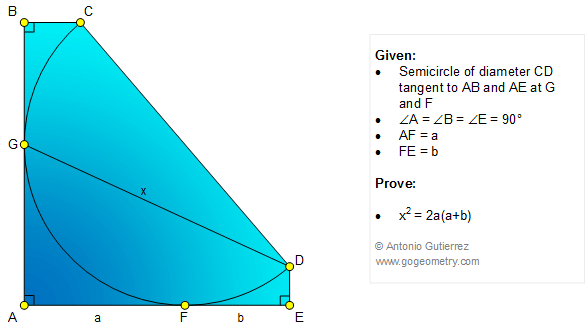# Online Geometry Problem 1083: Semicircle, Diameter, Perpendicular, 90 Degrees, Tangent Line, Metric Relations. Level: High School, SAT Prep, College, Mathematics Education

< PREVIOUS PROBLEM  |  NEXT PROBLEM >

 The figure below shows a semicircle of diameter CD tangent to AB and AE at G and F, respectively, so that angle A = angle B = angle E = 90 degrees. If AF = a, FE = b, and DG = x, prove that x2 = 2a(a+b).Geometry problem solving is one of the most challenging skills for students to learn. When a problem requires auxiliary construction, the difficulty of the problem increases drastically, perhaps because deciding which construction to make is an ill-structured problem. By “construction,” we mean adding geometric figures (points, lines, planes) to a problem figure that wasn’t mentioned as "given."

Home | SearchGeometry | Problems | All Problems | Open Problems | Visual Index | 10 Problems | 1081-1090 | Circle | Triangle | Diameter | Perpendicular linesTangent Line | Email | Solution / comment | by Antonio Gutierrez
Last updated: Feb 14, 2015